## 7.1.3 The Velocity-Tuning Method

An alternative to defining the problem in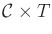is to decouple it into a path planning part and a motion timing part . Algorithms based on this method are not complete, but velocity tuning is an important idea that can be applied elsewhere. Suppose there are both stationary obstacles and moving obstacles. For the stationary obstacles, suppose that some path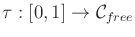has been computed using any of the techniques described in Chapters 5 and 6.

The timing part is then handled in a second phase. Design a timing function (or time scaling),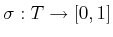, that indicates for time,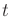, the location of the robot along the path,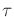. This is achieved by defining the composition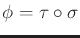, which maps from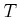tovia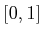. Thus,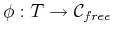. The configuration at time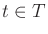is expressed as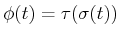.

A 2D state space can be defined as shown in Figure 7.4. The purpose is to convert the design of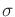(and consequently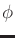) into a familiar planning problem. The robot must move along its path from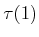towhile an obstacle,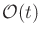, moves along its path over the time interval. Let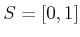denote the domain of. A state space,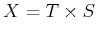, is shown in Figure 7.4b, in which each point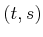indicates the timeand the position along the path,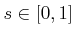. The obstacle region inis defined as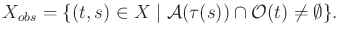(7.5)

Once again,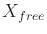is defined as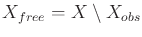. The task is to find a continuous path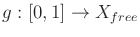. If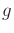is time-monotonic, then a position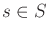is assigned for every time,. These assignments can be nicely organized into the timing function,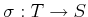, from whichis obtained byto determine where the robot will be at each time. Being time-monotonic in this context means that the path must always progress from left to right in Figure 7.4b. It can, however, be nonmonotonic in the positive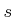direction. This corresponds to moving back and forth along, causing some configurations to be revisited.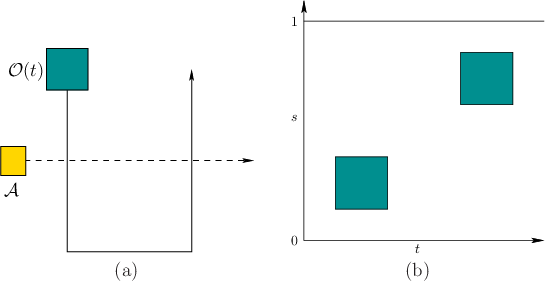Any of the methods described in Formulation 7.1 can be applied here. The dimension ofin this case is always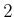. Note that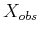is polygonal if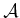and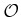are both polygonal regions and their paths are piecewise-linear. In this case, the vertical decomposition method of Section 6.2.2 can be applied by sweeping along the time axis to yield a complete algorithm (it is complete after having committed to, but it is not complete for Formulation 7.1). The result is shown in Figure 7.5. The cells are connected only if it is possible to reach one from the other by traveling in the forward time direction. As an example of a sampling-based approach that may be preferable whenis not polygonal, place a grid overand apply one of the classical search algorithms described in Section 5.4.2. Once again, only path segments inthat move forward in time are allowed.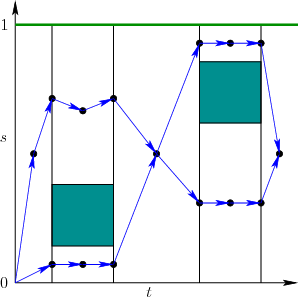Steven M LaValle 2020-08-14